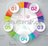# Verbal Reasoning - Number SeriesVerbal Reasoning – Number Series Aptitude Questions and Answers with solutions and Explanations for Bank PO, UPSC, CSAT, MBA, CAT, SSC-CGL, IBPS, Bank Clerical etc.

# Verbal Reasoning →Series Completion → Number Series→Question : 61

Find the missing term in the following series: 3, 8, 13, 24, 41, ? [A]70 [B]75 [C]80 [D]85 Show Answer 70 In the above question, the pattern followed is : nth term + (n + 1)th term + (n + 1) = (n +2)th term. Thus, 1st term + 2nd term + 2nd term = ..

# Verbal Reasoning →Series Completion → Number Series→Question : 60

Find the missing term in the following series: 66, 36, 18, ? [A]3 [B]6 [C]8 [D]9 Show Answer 8 In the above question, each term in the series is the product of the digits of the preceding term. So, missing term = 1 x 8 = 8.

# Verbal Reasoning →Series Completion → Number Series→Question : 59

Find the missing term in the following series: 1, 3, 4, 8, 15, 27, ? [A]37 [B]44 [C]50 [D]55 Show Answer 50 In the above question, the sum of any three consecutive terms of the series gives the next term. So, missing number = 8 + 15 + 27 = 50.

# Verbal Reasoning →Series Completion → Number Series→Question : 58

Find the missing term in the following series: 5824, 5242, ?, 4247, 3823 [A]4467 [B]4718 [C]4856 [D]5164 Show Answer 4718 In the above question, each term in the series is obtained by subtracting from the preceding term the number formed by the first three digits of the preceding term. So, missing term = 5242 – ..

# Verbal Reasoning →Series Completion → Number Series→Question : 57

Find the missing term in the following series: 589654237, 89654237, 8965423, 9654423, ? [A]58965 [B]65423 [C]89654 [D]96542 Show Answer 96542 In the above question, the digits are removed one by one from the beginning and the end in order alternately so as to obtain the subsequent terms of the series.